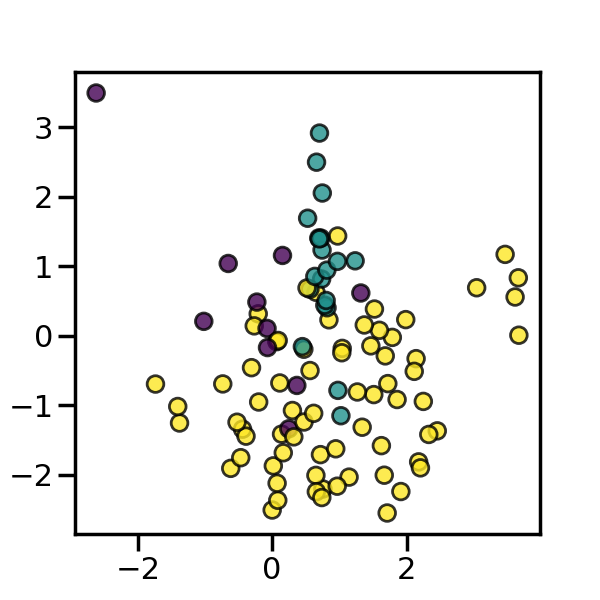# 4. Combination of over- and under-sampling#

We previously presented `SMOTE` and showed that this method can generate noisy samples by interpolating new points between marginal outliers and inliers. This issue can be solved by cleaning the space resulting from over-sampling.

In this regard, Tomek’s link and edited nearest-neighbours are the two cleaning methods that have been added to the pipeline after applying SMOTE over-sampling to obtain a cleaner space. The two ready-to use classes imbalanced-learn implements for combining over- and undersampling methods are: (i) `SMOTETomek` [BPM04] and (ii) `SMOTEENN` [BBM03].

Those two classes can be used like any other sampler with parameters identical to their former samplers:

```>>> from collections import Counter
>>> from sklearn.datasets import make_classification
>>> X, y = make_classification(n_samples=5000, n_features=2, n_informative=2,
...                            n_redundant=0, n_repeated=0, n_classes=3,
...                            n_clusters_per_class=1,
...                            weights=[0.01, 0.05, 0.94],
...                            class_sep=0.8, random_state=0)
>>> print(sorted(Counter(y).items()))
[(0, 64), (1, 262), (2, 4674)]
>>> from imblearn.combine import SMOTEENN
>>> smote_enn = SMOTEENN(random_state=0)
>>> X_resampled, y_resampled = smote_enn.fit_resample(X, y)
>>> print(sorted(Counter(y_resampled).items()))
[(0, 4060), (1, 4381), (2, 3502)]
>>> from imblearn.combine import SMOTETomek
>>> smote_tomek = SMOTETomek(random_state=0)
>>> X_resampled, y_resampled = smote_tomek.fit_resample(X, y)
>>> print(sorted(Counter(y_resampled).items()))
[(0, 4499), (1, 4566), (2, 4413)]
```

We can also see in the example below that `SMOTEENN` tends to clean more noisy samples than `SMOTETomek`.